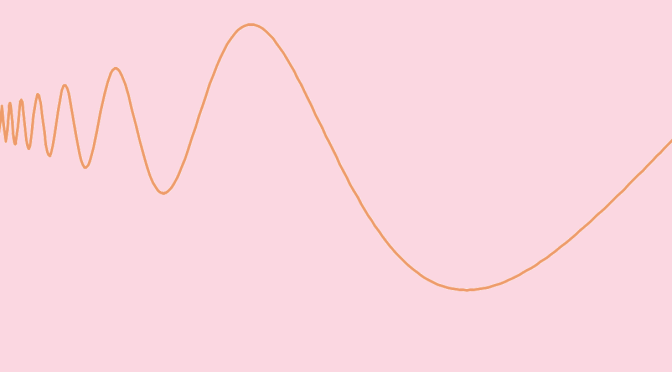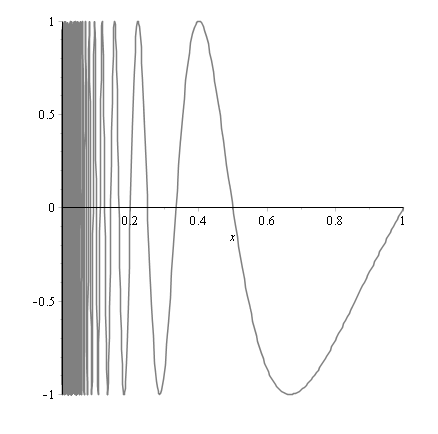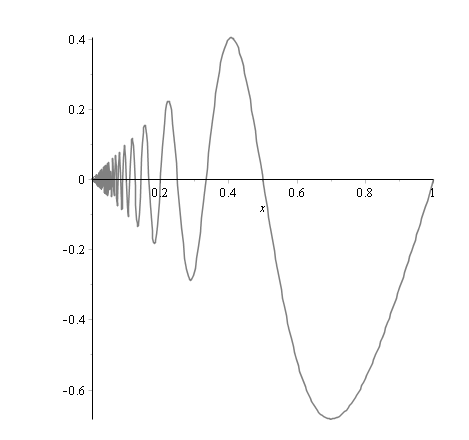# A continuous function which is not of bounded variation

## Introduction on total variation of functions

Recall that a function of bounded variation, also known as a BV-function, is a real-valued function whose total variation is bounded (finite).

Being more formal, the total variation of a real-valued function $$f$$, defined on an interval $$[a,b] \subset \mathbb{R}$$ is the quantity:
$V_a^b(f) = \sup\limits_{P \in \mathcal{P}} \sum_{i=0}^{n_P-1} \left\vert f(x_{i+1}) – f(x_i) \right\vert$ where the supremum is taken over the set $$\mathcal{P}$$ of all partitions of the interval considered.

## First example of a function which is not of bounded variationIt is easy to show a function that is not of bounded variation. Consider the function $$f$$ defined on the interval $$[0,1]$$ by:
$f(x)=\left\{ \begin{array}{ll} 0 & \mbox{if } x=0\\ \frac{1}{x} & \mbox{if } x \in (0,1]\\ \end{array} \right.$
For $$0 < u < 1$$, we have $$V_0^1(f) > V_u^1(f) = \frac{1}{u} – 1$$ and taking $$u$$ as small as desired we get $$V_0^1(f) = +\infty$$. The function $$f$$ is not bounded on its domain.

## A bounded function which is not of bounded variationCan a bounded function not be of bounded variation? The answer is also positive as proven by taking $$g$$ defined on the interval $$[0,1]$$ by:
$g(x)=\left\{ \begin{array}{ll} 0 & \mbox{if } x=0\\ \sin(\frac{\pi}{x}) & \mbox{if } x \in (0,1]\\ \end{array} \right.$
For an integer $$p \ge 1$$, we have $$V_0^1(g) > V_{\frac{1}{2p+1/2}}^{\frac{2}{5}}(g) = 4(p-1)$$ and therefore $$V_0^1(g) = +\infty$$.

## A continuous function which is not of bounded variationAnd finally we prove that the function $$h$$ defined on the interval $$[0,1]$$ by:
$h(x)= \left\{ \begin{array}{ll} 0 & \mbox{if } x=0\\ x \sin(\frac{\pi}{x}) & \mbox{if } x \in (0,1]\\ \end{array} \right.$
is continuous and not of bounded variation. Indeed $$h$$ is continuous at $$x \neq 0$$ as it is the product of two continuous functions at that point. $$h$$ is also continuous at $$0$$ because $$|h(x)| \le x$$ for $$x \in [0,1]$$. We now consider the total variation of $$h$$. For $$2 \le p$$ integer, we have $$V_0^1(h) \ge V_{\frac{1}{2p+1/2}}^{\frac{2}{5}}(h)$$ as $$[\frac{1}{2p+1/2},\frac{2}{5}] \subset [0,1]$$. Taking $$\{\frac{1}{2p+1/2},\frac{1}{2p-1/2},\frac{1}{2p-3/2},\dots,\frac{1}{2+1/2}=\frac{2}{5}\}$$ as a partition of $$[\frac{1}{2p+1/2},\frac{2}{5}]$$, one can prove that $$V_{\frac{1}{2p+1/2}}^{\frac{2}{5}}(h) \ge 2\sum_{i=1}^{p-1} \frac{1}{i+2}$$. As the last series diverges, $$h$$ is not of bounded variation.